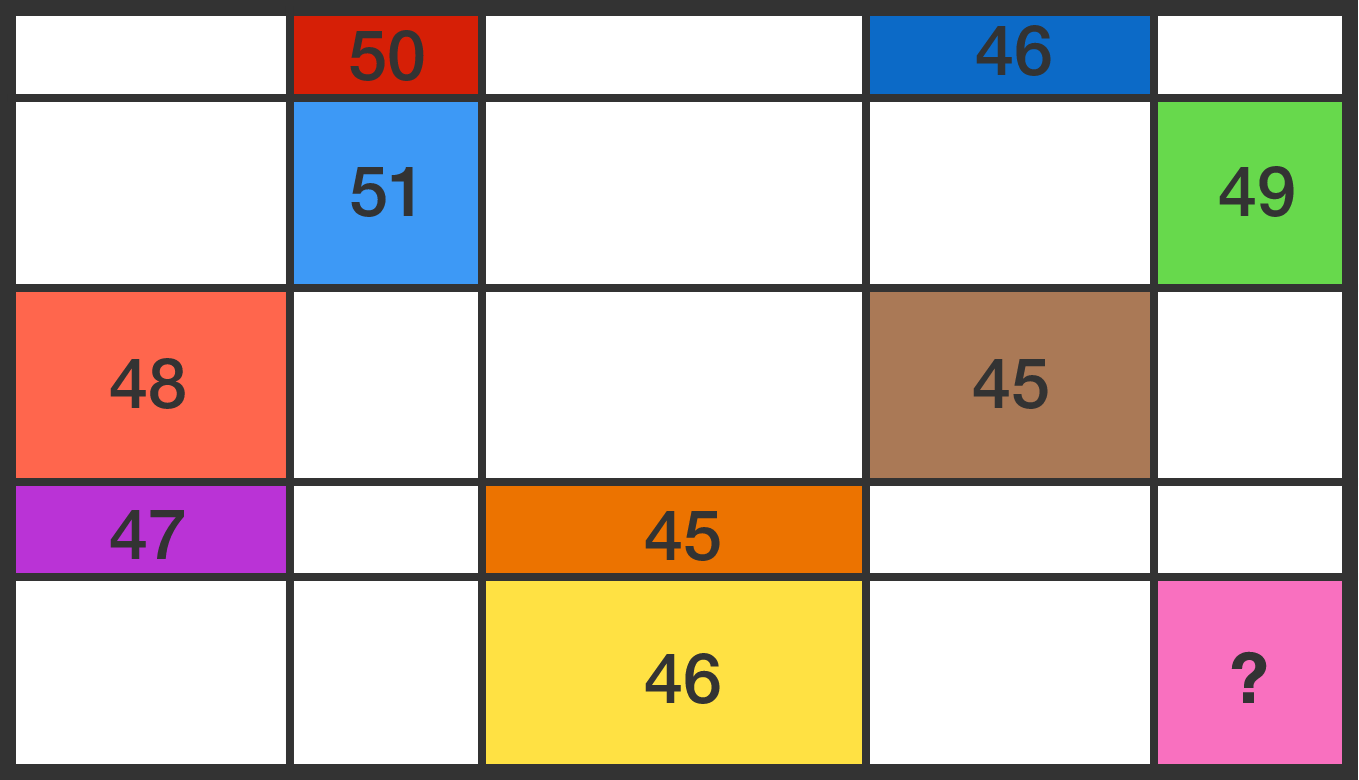# 25 rectangles

A rectangle is divided into 25 smaller rectangles, the perimeters of 9 of which are known to us by the numbers in the figure (which is not drawn to scale).

What is the perimeter of the pink rectangle?×